# Heap (Binary Heap)

Jan. 21, 2019 23567

Prerequisite - Binary Tree

A heap is a data structure which uses a binary tree for its implementation. It is the base of the algorithm heapsort and also used to implement a priority queue. It is basically a complete binary tree and generally implemented using an array. The root of the tree is the first element of the array.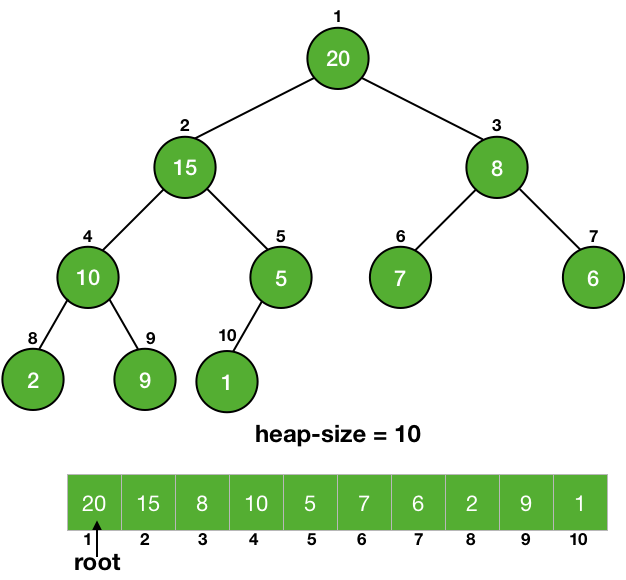Since a heap is a binary tree, we can also use the properties of a binary tree for a heap i.e.,

$Parent(i) = \lfloor \frac{i}{2} \rfloor$

$Left(i) = 2*i$

$Right(i) = 2*i + 1$

We declare the size of the heap explicitly and it may differ from the size of the array. For example, for an array with a size of Array.length, the heap will only contain the elements which are within the declared size of the heap.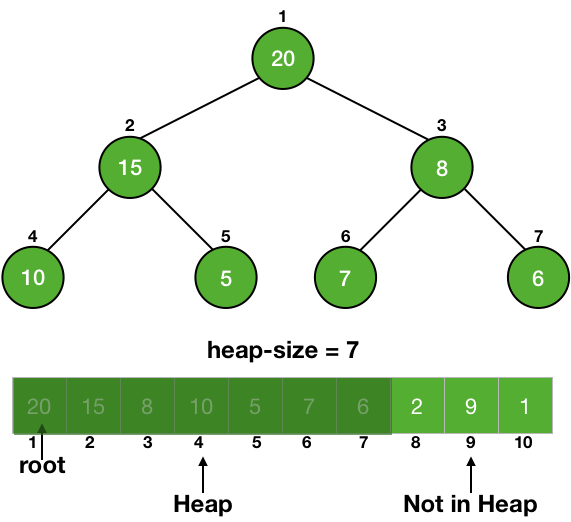## Properties of a heap

A heap is implemented using a binary tree and thus follow its properties but it has some additional properties which differentiate it from a normal binary tree. Basically, we implement two kind of heaps:

Max Heap →  In a max-heap, the value of a node is either greater than or equal to the value of its children.

A[Parent[i]] >= A[i] for all nodes i > 1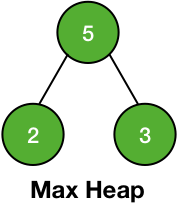Min Heap → The value of a node is either smaller than or equal to the value of its children

A[Parent[i]] <= A[i] for all nodes i > 1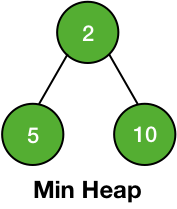Thus in max-heap, the largest element is at the root and in a min-heap, the smallest element is at the root.

Now, we know what is a heap, so let's focus on making a heap from an array and some basic operations done on a heap.

## Heapify

Heapify is an operation applied on a node of a heap to maintain the heap property. It is applied on a node when its children (left and right) are heap (follow the property of heap) but the node itself may be violating the property.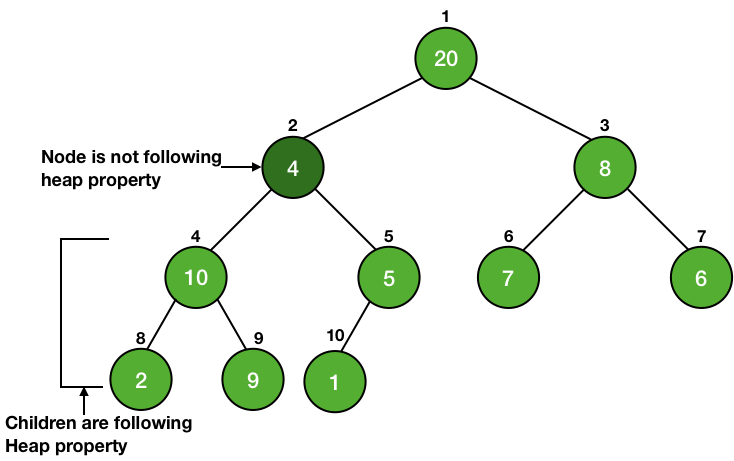We simply make the node travel down the tree until the property of the heap is satisfied. It is illustrated on a max-heap in the picture given below.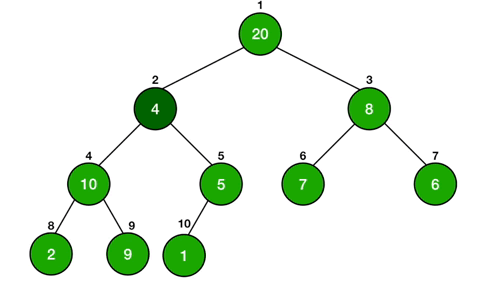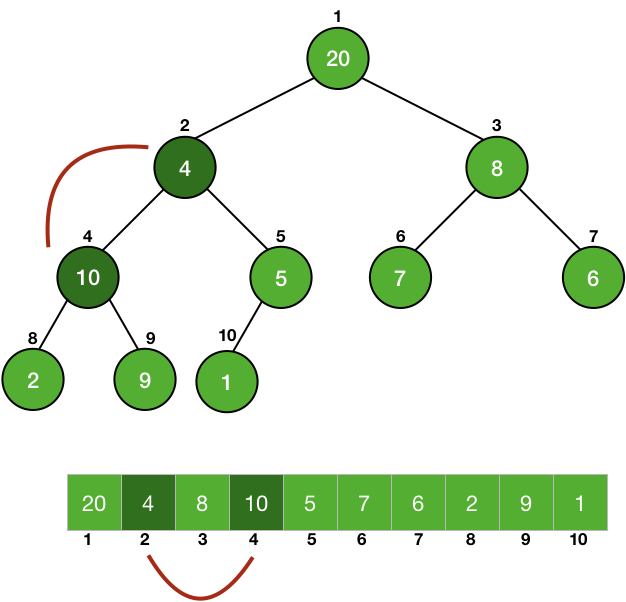We are basically swapping the node with the child having the larger value. By doing this, the node is now larger than its two children. You can see that the node 2 (value of 10) is now larger than its children 4 (value of 4) and 5 (value of 5).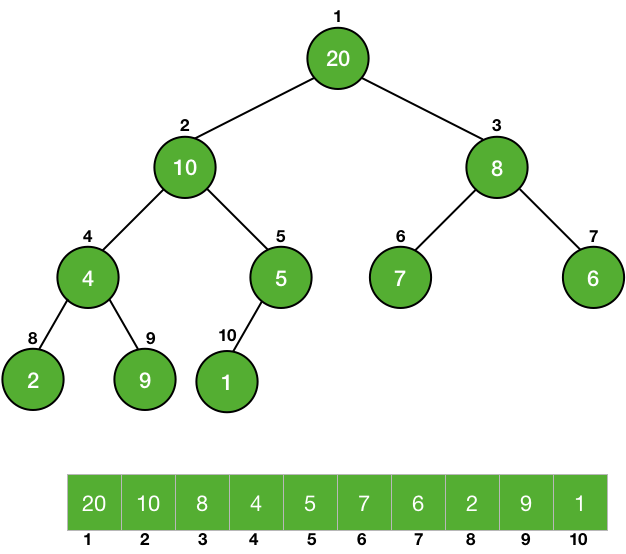But the child whose value was swapped might be violating the heap property. In the above picture, node 4 is smaller than the node 9 and thus, it is violating the max-heap property.

So, we are again implementing the Heapify operation on the child. This will be repeated until the property of max-heap is satisfied.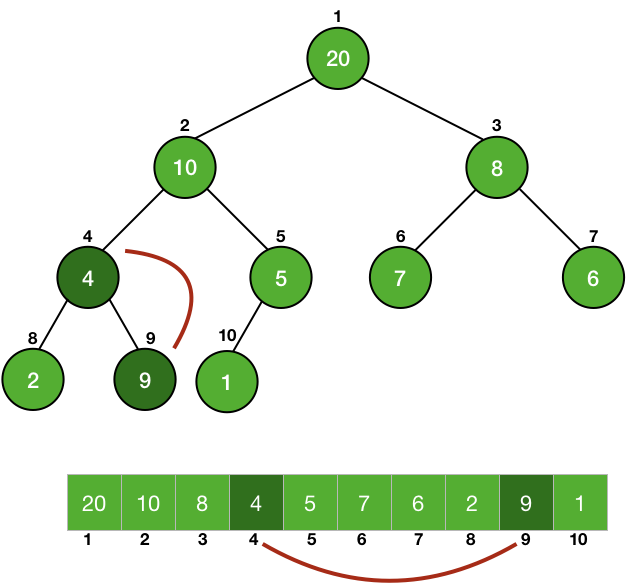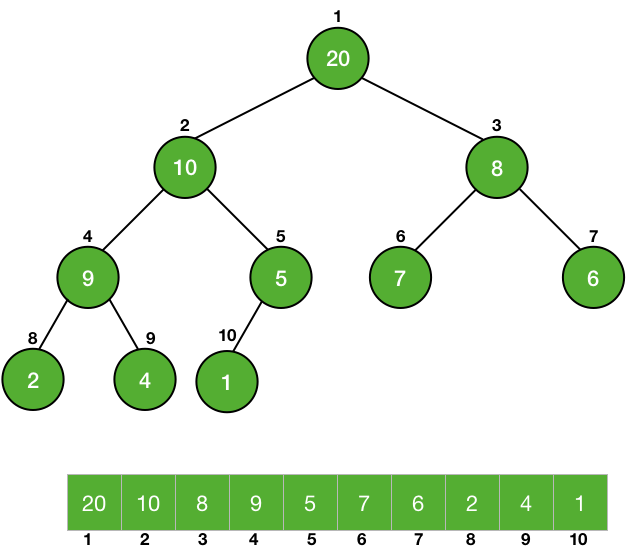You can see that after the completion of the Heapify operation, the tree is now a heap. So, let's look at the code to Heapify a max-heap

### Code for Max-Heapify

MAX-HEAPIFY(A, i)
left = 2i
right = 2i + 1

// checking for largest among left, right and node i
largest = i
if left <= heap_size
if (A[left] > A[largest])
largest = left

if right <= heap_size
if(A[right] > A[largest])
largest = right

if largest != i //node is not the largest, we need to swap
swap(A[i], A[largest])
MAX-HEAPIFY(A, largest) // chlid after swapping might be violating max-heap property


MAX-HEAPIFY(A, i) - A is the array used for the implementation of the heap and ‘ i’ is the node on which we are calling the function.

We are first calculating the largest among the node itself and its children.

Then, we are checking if the largest element is among its children - if largest != i. If the node itself is the largest, then the heap property is already satisfied but if it is not then we are swapping the largest element with the node - swap(A[i], A[largest]). As discussed earlier, the child whose value was swapped might not be following the heap property after the swapping, so we are again calling the function on it - MAX-HEAPIFY(A, largest).

Since the node on which we are applying Heapify is coming down and in the worst case, it may become a leaf. So, the worst-case running time will be the order of the height of the tree i.e., $O(\lg{n})$.

## Analysis of Heapify

Although we have predicted the running time to be $O(\lg{n})$, let's see it mathematically.

The calculations of left, right and maximum element are going to take $\Theta(1)$ time.

Now, we are left with the calculation of the time that will be taken by the MAX-HEAPIFY(A, largest) and it will depend on the size of the input.

The tree is divided into two subtrees. Since MAX-HEAPIFY is dependent on the size of the tree (or subtree in recursive calls), in the worst case, this size will be maximum. This will happen when the last level of the tree will be half full.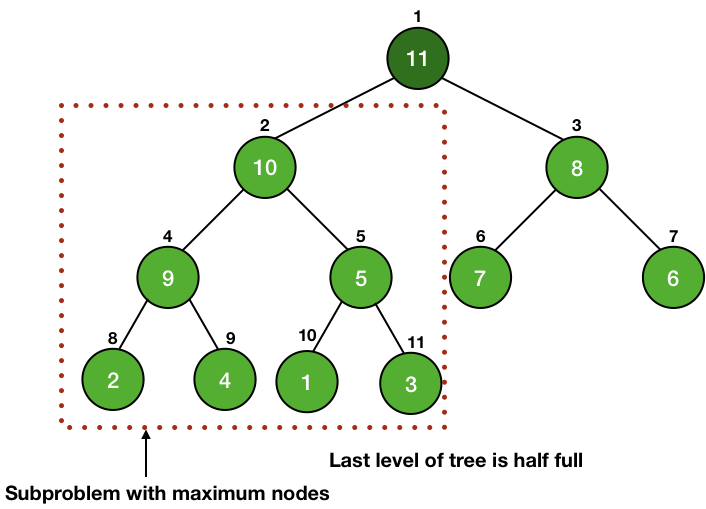In this case, one of the subtrees will have one level more than the other one. This will maximize the number of nodes in the subtree for a fixed number of nodes n in the complete binary tree.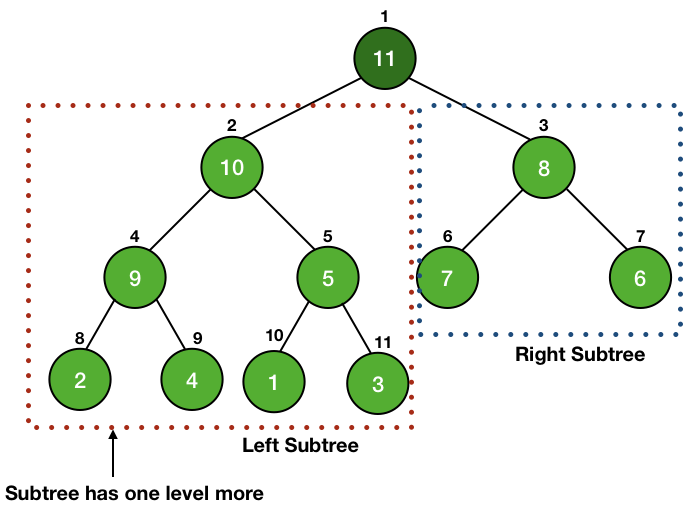We know that a tree with $i$ levels has a total number of $2^{i+1} - 1$. Thus, if the right subtree has $i$ levels, it will have $2^{i+1} - 1$ nodes and the left subtree will have $i+1$ levels and thus a total number of $2^{i+2} - 1$ nodes.

The total number of nodes in the tree $= 2^{i+1} - 1 + 2^{i+2} - 1 + 1(root) = n$

$2^{i+1} - 1 + 2^{i+2} = n$

$2*2^i + 4*2^i = n+1$

$6*2^i = n+1$

$i =\lg{\frac{n+1}{6}}$

Now, the total number of nodes in the left subtree = $2^{i+2} - 1 = 4*2^i - 1 = \frac{4(n+1)}{6} - 1 = \frac{2(n+1)}{3} -1 = \frac{2n}{3} - \frac{1}{3}$

$\frac{2n}{3} - \frac{1}{3} \le \frac{2n}{3}$

So, we can use $\frac{2n}{3}$ as its upper bound and write the recurrence equation as $T(n) \leq T(\frac{2n}{3}) + \Theta(1)$

By using Master's theorem, we can easily find out the running time of the algorithm to be $O(\lg{n})$.

We are left with one final task, to make a heap by the array provided to us. We know that Heapify when applied to a node whose children are heaps, makes the node also a heap. The leaves of a tree don't have any children, so they follow the property of a heap and are already heap.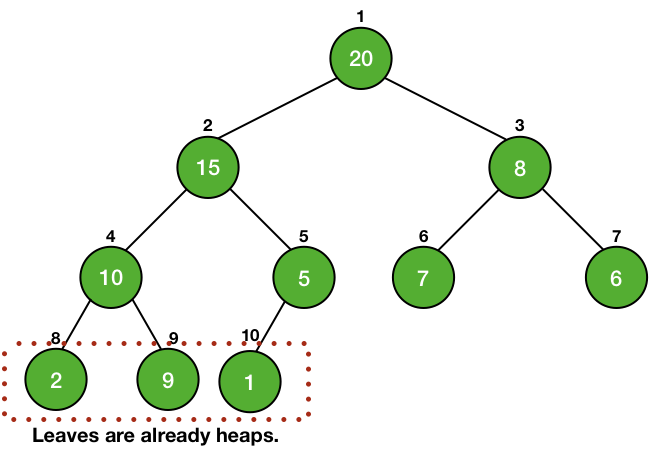We can implement the Heapify operation on the parent of these leaves to make them heaps.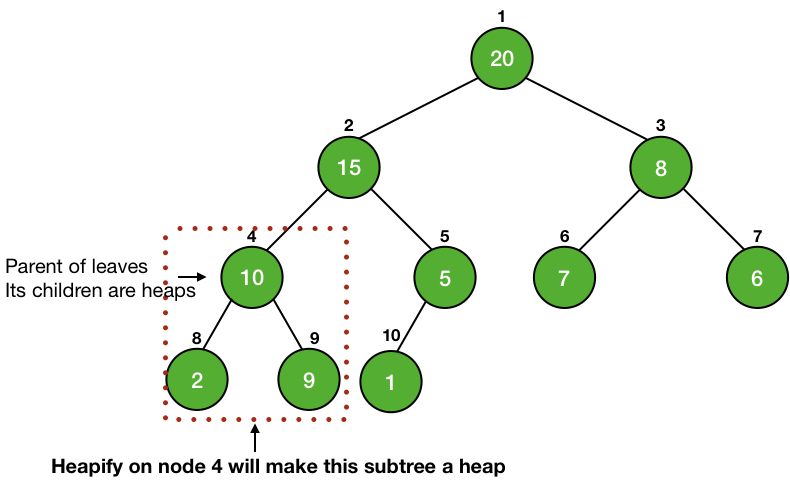We can simply iterate up to root and use the Heapify operation to make the entire tree a heap.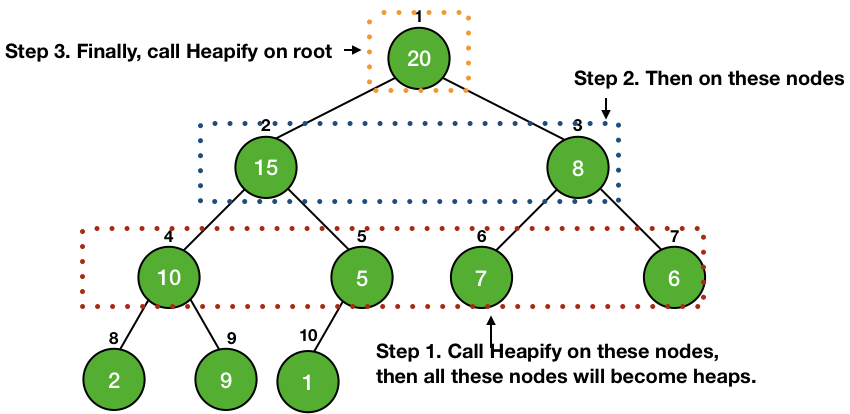### Code for Build-Heap

We simply have to iterate from the parent of the leaves to the root of the tree to call Heapify on each node. For this, we need to find the leaves of the tree. The nodes from $\lceil \frac{n}{2}\rceil + 1$ to $n$ are leaves. We can easily check this because $2*i = 2 * ( \lceil \frac{n}{2}\rceil + 1 ) = n+2$ which is outside the heap and thus, this node doesn't have any children, so it is a leaf. Thus, we can make our iteration from $\lceil\frac{n}{2}\rceil$ to root and call the Heapify operation.

BUILD-HEAP(A)
for i in floor(A.length/2) downto 1
MAX-HEAPIFY(A, i)


## Analysis of Build-Heap

We know that the Heapify takes $O(\lg{n})$ time and there are $O(n)$ such calls. Thus a total of $O(n\lg{n})$ time.

This gives us an upper bound for our operation but we can reduce this upper bound and get a more precise running time of $O(n)$.

### A More Precise Analysis

We know that the Heapify makes a node travel down the tree, so it will take $O(h)$ time, where h is the height of the node.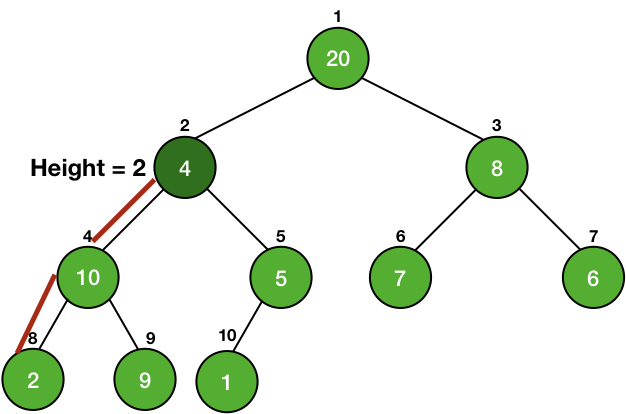We also know that the height of a node is $O(\lg{n})$, where n is the number of nodes in the subtree.

Also, the maximum number of nodes with a height h is $\lceil \frac{n}{2^{h+1}}\rceil$ (You can prove it by induction).

So, the total time taken by the Heapify function for all nodes at a height h = $O(h)*\lceil \frac{n}{2^{h+1}}\rceil$ (height of the nodes*number of nodes).

Now, this height will change from $0$ to $\lfloor \lg{n}\rfloor$.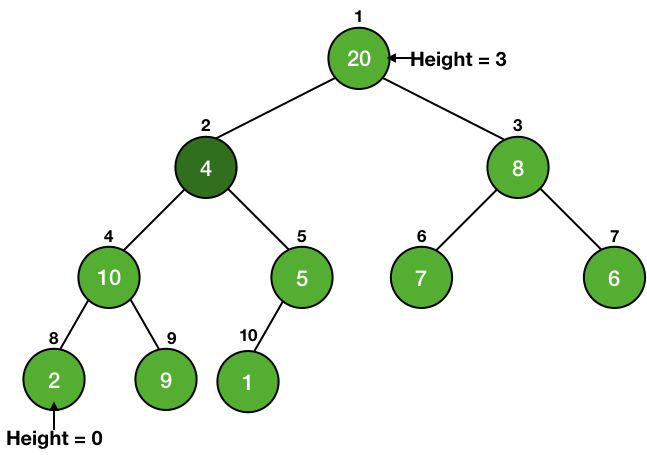Thus, the total time taken for all nodes = $$\sum_{h=0}^{\lfloor \lg{n}\rfloor} {\left( \Bigl\lceil \frac{n}{2^{h+1}}\Bigr\rceil *O(h) \right)}$$

$$= O \left( n * \sum_{h=0}^{\lfloor \lg{n}\rfloor} {\Bigl\lceil \frac{h}{2*2^{h}}}\Bigr\rceil \right)$$

$$= O \left( n * \sum_{h=0}^{\lfloor \lg{n}\rfloor} {\Bigl\lceil \frac{h}{2^{h}}}\Bigr\rceil \right)$$

Taking the term $\sum_{h=0}^{\lfloor \lg{n}\rfloor} {\Bigl\lceil \frac{h}{2^{h}}}\Bigr\rceil$.

$$\sum_{h=0}^{\lfloor \lg{n}\rfloor} {\Bigl\lceil \frac{h}{2^{h}}}\Bigr\rceil \lt \sum_{h=0}^{\infty} {\Bigl\lceil \frac{h}{2^{h}}}\Bigr\rceil$$

$$\text{Let }S = \sum_{h=0}^{\infty} {\Bigl\lceil \frac{h}{2^{h}}}\Bigr\rceil$$

$$\text{or, }S = 1 + \frac{1}{2} + \frac{2}{2^2} + \frac{3}{2^3} + ....$$

$$2S = 2 + 1 + \frac{2}{2} + \frac{3}{2^2} + \frac{4}{2^3} + ....$$

$2S - S$,

$$2S = 2 + 1 + \frac{2}{2} + \frac{3}{2^2} + \frac{4}{2^3} + ....$$ $$S = 0 + 1 + \frac{1}{2} + \frac{2}{2^2} + \frac{3}{2^3} ....$$ $$2S - S = S = 2 + 0 + \frac{1}{2} + \frac{1}{2^2} + \frac{1}{2^3} + ...$$

The above equation is an infinite G.P. as $\frac{1}{2}$ as the first term as well as the common ratio.

$$S = 2 + \frac{\frac{1}{2}}{1 - \frac{1}{2}} = 2$$

So, $S = \sum_{h=0}^{\lfloor \lg{n}\rfloor} {\Bigl\lceil \frac{h}{2^{h}}}\Bigr\rceil = 2$.

Putting this value in $O \left( n * \sum_{h=0}^{\lfloor \lg{n}\rfloor} {\Bigl\lceil \frac{h}{2^{h}}}\Bigr\rceil \right)$.

Running Time = $O\left(n * 2\right) = O(n)$

So, we can make a heap from an array in a linear time.

## Code for Heap - C, Java and Python

• C
• Python
• Java
#include <stdio.h>

int tree_array_size = 11;
int heap_size = 10;

void swap( int *a, int *b ) {
int t;
t = *a;
*a = *b;
*b = t;
}

//function to get right child of a node of a tree
int get_right_child(int A[], int index) {
if((((2*index)+1) < tree_array_size) && (index >= 1))
return (2*index)+1;
return -1;
}

//function to get left child of a node of a tree
int get_left_child(int A[], int index) {
if(((2*index) < tree_array_size) && (index >= 1))
return 2*index;
return -1;
}

//function to get the parent of a node of a tree
int get_parent(int A[], int index) {
if ((index > 1) && (index < tree_array_size)) {
return index/2;
}
return -1;
}

void max_heapify(int A[], int index) {
int left_child_index = get_left_child(A, index);
int right_child_index = get_right_child(A, index);

// finding largest among index, left child and right child
int largest = index;

if ((left_child_index <= heap_size) && (left_child_index>0)) {
if (A[left_child_index] > A[largest]) {
largest = left_child_index;
}
}

if ((right_child_index <= heap_size && (right_child_index>0))) {
if (A[right_child_index] > A[largest]) {
largest = right_child_index;
}
}

// largest is not the node, node is not a heap
if (largest != index) {
swap(&A[index], &A[largest]);
max_heapify(A, largest);
}
}

void build_max_heap(int A[]) {
int i;
for(i=heap_size/2; i>=1; i--) {
max_heapify(A, i);
}
}

int main() {
//tree is starting from index 1 and not 0
int A[] = {0, 15, 20, 7, 9, 5, 8, 6, 10, 2, 1};
build_max_heap(A);
int i;
for(i=1; i<=heap_size; i++) {
printf("%d\n",A[i]);
}
return 0;
}


heap_size = 10

# function to get right child of a node of a tree
def get_right_child(A, index):
if((((2*index)+1) < len(A)) and (index >= 1)):
return (2*index)+1
return -1

# function to get left child of a node of a tree
def get_left_child(A, index):
if(((2*index) < len(A)) and (index >= 1)):
return 2*index
return -1

# function to get the parent of a node of a tree
def get_parent(A, index):
if ((index > 1) and (index < len(A))):
return index//2
return -1

def max_heapify(A, index):
left_child_index = get_left_child(A, index)
right_child_index = get_right_child(A, index)

# finding largest among index, left child and right child
largest = index

if ((left_child_index <= heap_size) and (left_child_index>0)):
if (A[left_child_index] > A[largest]):
largest = left_child_index

if ((right_child_index <= heap_size and (right_child_index>0))):
if (A[right_child_index] > A[largest]):
largest = right_child_index

# largest is not the node, node is not a heap
if (largest != index):
A[index], A[largest] = A[largest], A[index]
max_heapify(A, largest)

def build_max_heap(A):
for i in range(heap_size//2, 0, -1):
max_heapify(A, i)

if __name__ == '__main__':
# tree is starting from index 1 and not 0
A = [0, 15, 20, 7, 9, 5, 8, 6, 10, 2, 1]
build_max_heap(A)
print(A[1:heap_size+1])


class Heap {
public static int heapSize = 10;

//function to get right child of a node of a tree
public static int getRightChild(int A[], int index) {
if((((2*index)+1) < A.length && (index >= 1)))
return (2*index)+1;
return -1;
}

//function to get left child of a node of a tree
public static int getLeftChild(int A[], int index) {
if(((2*index) < A.length && (index >= 1)))
return 2*index;
return -1;
}

//function to get the parent of a node of a tree
public static int getParent(int A[], int index) {
if ((index > 1) && (index < A.length)) {
return index/2;
}
return -1;
}

public static void maxHeapify(int A[], int index) {
int leftChildIndex = getLeftChild(A, index);
int rightChildIndex = getRightChild(A, index);

// finding largest among index, left child and right child
int largest = index;

if ((leftChildIndex <= heapSize) && (leftChildIndex>0)) {
if (A[leftChildIndex] > A[largest]) {
largest = leftChildIndex;
}
}

if ((rightChildIndex <= heapSize && (rightChildIndex>0))) {
if (A[rightChildIndex] > A[largest]) {
largest = rightChildIndex;
}
}

// largest is not the node, node is not a heap
if (largest != index) {
int temp;
//swapping
temp = A[largest];
A[largest] = A[index];
A[index] = temp;
maxHeapify(A, largest);
}
}

public static void buildMaxHeap(int A[]) {
int i;
for(i=heapSize/2; i>=1; i--) {
maxHeapify(A, i);
}
}

public static void main(String[] args) {
//tree is starting from index 1 and not 0
int A[] = {0, 15, 20, 7, 9, 5, 8, 6, 10, 2, 1};
buildMaxHeap(A);
for(int i=1; i<=heapSize; i++) {
System.out.println(A[i]);
}
}
}



Liked the post?
Developer and founder of CodesDope.
0 COMMENT